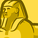# QlikView App Dev

Discussion Board for collaboration related to QlikView App Development.

Announcements
Support Cases coming to Qlik Community Oct. 4! Start chats, open cases, explore resources: READ DETAILS
cancel
Showing results for
Did you mean:Creator II

## Merge two values to create one in Dimension

I have a Calculated Dimension as below:

=if(vFlag=1,
if(Response = '1', 'A',
if(Response = '2', 'B',

if(Response = '3, 'C',

if(Response = '4', 'D'))))

I need to merge Responses 3 and 4 and show them as 'D'.

I am using this but it does not work

if(pick(Match(Response, '3','4'), 4,3)=1,'D'

I have also tried if(Response='3' or '4','D') but still doesn't work.

Labels (3)

• ### Merge two values

3 Solutions

Accepted SolutionsMVP

Try this

``````=if(vFlag=1,
if(Response = '1', 'A',
if(Response = '2', 'B',
if(Response = '3', 'D',
if(Response = '4', 'D'))))``````

or

``````=if(vFlag=1,
if(Response = '1', 'A',
if(Response = '2', 'B',
if(Match(Response, '3', '4'), 'D'))))``````Partner

if(match(Response,'3','4'),D)MVP

Change the expression to this

``Count({<Response = {"=Len(Response) > 0"}>} Response)``
5 RepliesMVP

Try this

``````=if(vFlag=1,
if(Response = '1', 'A',
if(Response = '2', 'B',
if(Response = '3', 'D',
if(Response = '4', 'D'))))``````

or

``````=if(vFlag=1,
if(Response = '1', 'A',
if(Response = '2', 'B',
if(Match(Response, '3', '4'), 'D'))))``````Partner

if(match(Response,'3','4'),D)Creator II
Author

Hi @sunny_talwar  I have used both but the issue is, I have an expression in the chart

(if(Len(Response)>0, count(Response)))

This only gives me two Dimensions A and B but not D. When I remove the expression and create a dummy empty expression, I am able to see A, B, and D. Am I doing something wrong here? The Expression is the issue.MVP

Change the expression to this

``Count({<Response = {"=Len(Response) > 0"}>} Response)``Creator II
Author

Awesome, Savior you are 🙂Community Browser